Question
22 views

For the hypothetical reaction A → products, the concentration of A was monitored over time. From the following graph, what is the rate constant for the decomposition of A?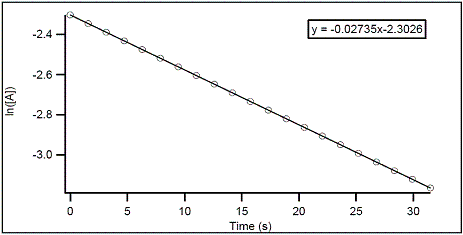help_outlineImage Transcriptionclosey = -0.02735x-2.3026 -2.4 -2.6 -2.8 -3.0 10 15 20 25 30 Time (s) In((A]) fullscreen
check_circle

Step 1

From the given data,

The graph between ln [A]  vs  time is a straight line.

This indicates that the given hypothetical reaction is of first order.

Rate of reaction :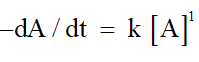The rate equation obtained from the above rate law is: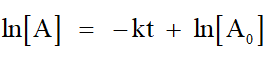Which is in the form of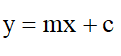By comparing: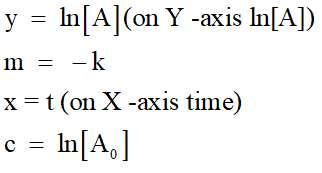Step 2

The given equation is:

y = - 0.02735 x - 2.3026

which is in the form of y =mx +c

The slope of the line m = -0.02735

We know that : m=-k

so,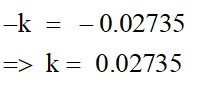...

### Want to see the full answer?

See Solution

#### Want to see this answer and more?

Solutions are written by subject experts who are available 24/7. Questions are typically answered within 1 hour.*

See Solution
*Response times may vary by subject and question.
Tagged in